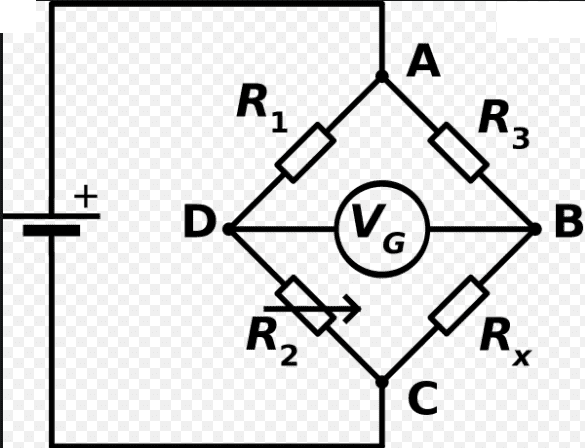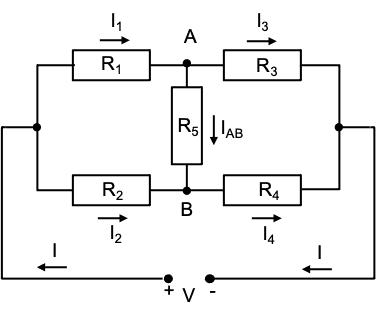# How to do the calculations for a Wheatstone Bridge

songokuI know at balanced condition, the current flowing through galvanometer = 0 and R1 . Rx = R2 . R3

What I want to ask is how to analyze the circuit until we can conclude the current will be zero. I have learned about Kirchhoff's 1st and 2nd law and also about potential divider. I am guessing there is something to do with potential divider. By changing the value of R2, we can get VD = VB so no current flowing through galvanometer but how to do the calculation / analysis? Can we do it by using Kirchhoff's 1st and 2nd law and potential divider or we need something else beyond high school scope?

Thanks

Last edited by a moderator:

All you need is Ohm's law. Take the voltage at point C to be 0, and the voltage at point A to be V, which is the voltage supplied by the battery. Some current I3 flows through R3. So the voltage at point B is VB=V - I3*R3. The current I3 all flows through Rx, since no current flows through the galvanometer. So the current at point B is also VB=I3*Rx. Eliminating I3 from these two equations gives that VB=V*Rx/(R3+Rx). Similarly on the other side, VD=V*R2/(R1+R2). When VB=VD, you can solve for Rx=R2*R3/R1.

songoku
All you need is Ohm's law. Take the voltage at point C to be 0, and the voltage at point A to be V, which is the voltage supplied by the battery. Some current I3 flows through R3. So the voltage at point B is VB=V - I3*R3. The current I3 all flows through Rx, since no current flows through the galvanometer. So the current at point B is also VB=I3*Rx. Eliminating I3 from these two equations gives that VB=V*Rx/(R3+Rx). Similarly on the other side, VD=V*R2/(R1+R2). When VB=VD, you can solve for Rx=R2*R3/R1.
Sorry I think I don't explain myself clearly. I mean how we can get VB = VD, not how to get Rx . R1 = R2 . R3

Thanks

Gold Member
For the out of balance case, there are a couple of choices. In one you assume that you have a very high impedance voltmeter (much larger than the other resistors), then you just calculated the voltages with two independent voltage dividers, as above.

For the more general case you can put an impedance in place of the galvanometer. You can then solve this circuit by labeling all of the branch currents (6 of them) and write a set of quite simple equations for the currents from KVL and KCL. It is a 6x6 matrix, but the elements are simple.

Sorry I think I don't explain myself clearly. I mean how we can get VB = VD, not how to get Rx . R1 = R2 . R3

Thanks
That's how the bridge works. You adjust the variable resistor R2 until the galvanometer reads 0. Maybe I don't understand your question.

2022 Award
Sorry I think I don't explain myself clearly. I mean how we can get VB = VD, not how to get Rx . R1 = R2 . R3

Thanks
If ##V_B\neq V_D## then there is a potential difference between ##B## and ##D## and current flows one way or another through the galvanometer. We balance the bridge so that no current flows, so we know ##V_B=V_D##.

Homework Helper
Gold Member
I suspect OP wants to start from the general case, that is replace the galvanometer with a resistor, find the current in that resistor using Kirchhoff rules and then see under what conditions that current is zero.

•nasu, sophiecentaur and vanhees71
Gold Member
I mean how we can get VB = VD,
You may be looking at this in the 'wrong order'. The point about the condition VB = VD is that you have to adjust the resistors to achieve it. The values of the Rs will then fit the equation Rx . R1 = R2 . R3

You could say that VB=VD is an imposed condition.

songoku
That's how the bridge works. You adjust the variable resistor R2 until the galvanometer reads 0. Maybe I don't understand your question.
If ##V_B\neq V_D## then there is a potential difference between ##B## and ##D## and current flows one way or another through the galvanometer. We balance the bridge so that no current flows, so we know ##V_B=V_D##.
I am sorry. My question is just like what kuruman said (although the idea of changing galvanometer with a resistor did not cross my mind)
I suspect OP wants to start from the general case, that is replace the galvanometer with a resistor, find the current in that resistor using Kirchhoff rules and then see under what conditions that current is zero.

For the out of balance case, there are a couple of choices. In one you assume that you have a very high impedance voltmeter (much larger than the other resistors), then you just calculated the voltages with two independent voltage dividers, as above.

For the more general case you can put an impedance in place of the galvanometer. You can then solve this circuit by labeling all of the branch currents (6 of them) and write a set of quite simple equations for the currents from KVL and KCL. It is a 6x6 matrix, but the elements are simple.

Let say I change galvanometer to R5 and Rx to R4. I am thinking of stating I5 (current passing through R5) in terms of R1 to R5 then find what the condition is for I5 = 0

Can that be done by using KCL and KVL? I think I can write down three equations of KVL by using three different loops and maybe three equations using KCL (something like I1 = I2 + I5 , I4 = I3 + I5). By using algebra, can I state I5 in terms of R1 to R5 using this method? I have to deal with a lot of variables and it seems impossible to do that.

You may be looking at this in the 'wrong order'. The point about the condition VB = VD is that you have to adjust the resistors to achieve it. The values of the Rs will then fit the equation Rx . R1 = R2 . R3

You could say that VB=VD is an imposed condition.
I understand now it is an imposed condition. For balanced wheatstone bridge, it means current through galvanometer = 0 so it implies that VB = VD. Now I am looking for something more general about wheatstone bridge.

I apologize to everyone for not stating my question clearly.

Thanks

Gold Member
I have to deal with a lot of variables and it seems impossible to do that.
Not impossible. Maybe a little tedious. If you've solved systems of linear equations it will be familiar. Linear algebra is your friend here, but it's not necessary. You'll get one equation for every loop and one equation for every node; 7 equations, but only 6 unknown currents.

I understand now it is an imposed condition. For balanced wheatstone bridge, it means current through galvanometer = 0 so it implies that VB = VD.
@songoku , I think there is still a point that you are missing. An ideal voltmeter (galvanometer) has infinite resistance, so the current through the galvanometer is always zero, even if VB ≠ VD.

Gold Member
I have to deal with a lot of variables and it seems impossible to do that.
Now I am looking for something more general about wheatstone bridge.
If you can understand how do do the calculation for two or three resistors then there is no shame in reaching for a circuit analysis application do do it for more (hundreds, even). It's just a tool.•vanhees71
songoku
Not impossible. Maybe a little tedious. If you've solved systems of linear equations it will be familiar. Linear algebra is your friend here, but it's not necessary. You'll get one equation for every loop and one equation for every node; 7 equations, but only 6 unknown currents.
Ok I will try it again. I have not gotten 7 equations, only 5 equations so I am missing something

@songoku , I think there is still a point that you are missing. An ideal voltmeter (galvanometer) has infinite resistance, so the current through the galvanometer is always zero, even if VB ≠ VD.
I thought galvanometer is ammeter, for measuring current so ideal galvanometer will have zero resistance. So galvanometer is actually voltmeter?

If you can understand how do do the calculation for two or three resistors then there is no shame in reaching for a circuit analysis application do do it for more (hundreds, even). It's just a tool.At first I wonder whether I need knowledge beyond what I know right now to do what I ask in this thread but it seems I just need to use KCL and KVL and the problem is my algebra skill is lacking.

Thanks

I thought galvanometer is ammeter, for measuring current so ideal galvanometer will have zero resistance. So galvanometer is actually voltmeter?
You are correct that a galvanometer is an ammeter and ideally has zero resistance. Ignore my comment. I though a Wheatstone bridge typically had a voltmeter in the middle.

Homework Helper
Gold Member
Ok I will try it again. I have not gotten 7 equations, only 5 equations so I am missing something
You are not missing anything. Starting with the diagram shown below, write 3 Kirchhoff voltage and two node current equations which will give you a system of 5 equations and 5 unknowns, the currents. I don't count the total current as unknown since ##I=I_1+I_2=I_3+I_4.##

The two current equations that I used are ##I_1+I_2=I_3+I_4## and ##I_1=I_3+I_{\text{AB}}##.For the 3 voltage loops equations, be sure none of them is the sum of the other two. Good luck with solving the system.
Disclaimer
The system is one of those things that one has to do at least once in one's life. I have already done it many years ago, so I used Mathematica to get the solution. However, the answer for ##I_{\text{AB}}## makes eminent sense in retrospect.

•DaveE
songoku
For the 3 voltage loops equations, be sure none of them is the sum of the other two.
This is where my mistake is. I will try doing it again

Thank you very much for all the help and explanation phyzguy, DaveE, Ibix, kuruman, sophiecentaur

•sophiecentaur